# I.E. Irodov Solutions on Polarization of Light

Students can find the solutions of the Problems In General Physics I.E. Irodov - Polarization of Light on this page. A phenomenon caused due to the wave nature of electromagnetic radiation is known as Polarization in Physics. Students can expect one question from this topic for the entrance exams. Malus' law, Brewster's law, degree of polarization of light, Fresnel equations for the intensity of light reflected at the boundary between two dielectrics, natural and magnetic rotation of the plane of polarization etc, are the main points in this topic.

Students are recommended to go through these solutions so that they can be familiar with the type of questions from polarization of light.

### I.E. Irodov Solutions on Polarization of Light

1. A plane monochromatic wave of natural light with intensity I0 falls normally on a screen composed of two touching Polaroid half-planes. The principal direction of one Polaroid is parallel, and of the other perpendicular, to the boundary between them. What kind of diffraction pattern is formed behind the screen? What is the intensity of light behind the screen at the points of the plane perpendicular to the screen and passing through the boundary between the Polaroids?

Solution:

1.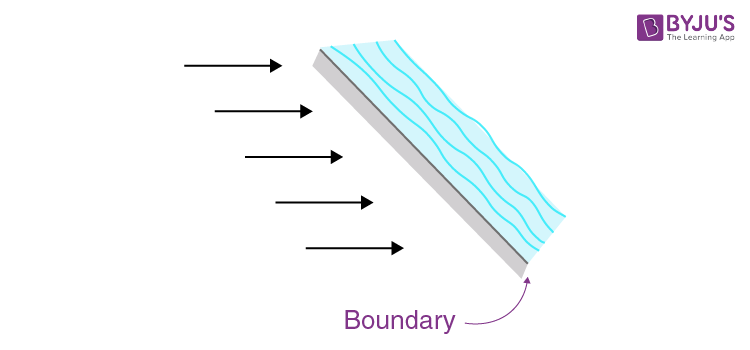Natural light can be considered to be an incoherent mixture of two plane-polarized light of intensity l0/2 with mutually perpendicular planes of vibration. The screen consisting of the two polaroid half-planes acts as an opaque half-screen for one or the other of these light waves. The resulting diffraction pattern has the alterations in intensity (in the illuminated region ) characteristic of a straight edge on both sides of the boundary. At the boundary the intensity due to either component is (I0/2)/4 and the total intensity is I0/2. (Recall that when the light of intensity I0 is incident on a straight edge, the illuminance in front of the edge is l0/4) .

2. A plane monochromatic wave of natural light with intensity Iofalls normally on an opaque screen with round hole corresponding to the first Fresnel zone for the observation point P. Find the intensity of light at the point P after the hole was covered with two identical Polaroids whose principal directions are mutually perpendicular and the boundary between them passes (a) along the diameter of the hole; (b) along the circumference of the circle limiting the first half of the Fresnel zone.

Solution:

1.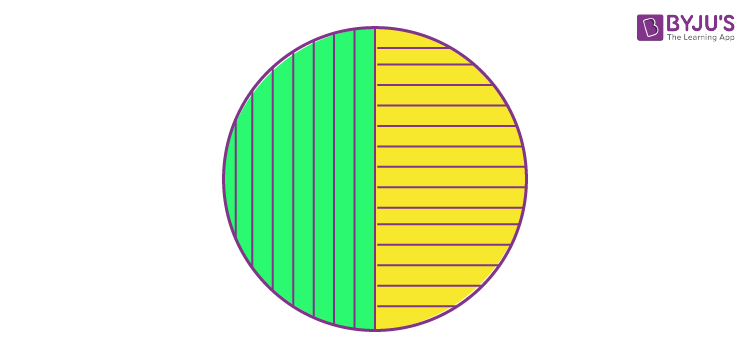(a) Assume first that there is no polaroid and the amplitude due to the entire hole which extends over the first Fresnel zone is A1. Then, we know, as usual, I0 = A12/4

When the Polaroid is introduced as shown above, each half transmits only the corresponding polarized light. If the full hole were covered by one polaroid the amplitude transmitted will be A1/√2. Therefore the amplitude transmitted in the present case will be A1/2√2 through either half. Since these transmitted waves are polarized in mutually perpendicular planes, the total intensity will be (A1/2√2)2 + (A1/2√2)2 = A12/4 = I0

(b) We interpret the problem to mean that the two polaroid pieces are separated along the circumference of the circle limiting the first half of the Fresnel zone. (This however is inconsistent with the polaroid’s being identical in shape; however, no other interpretation makes sense.) From (5 .103) and the previous problems we see that the amplitudes of the waves transmitted through the two parts is

(A1/2√2)(1 + i) and (A1/2√2)(1 - i) and the intensity is |(A12/2√2)(1 + i)|2 + |(A1/2√2)(1 - i)|2

= A12/2

= 2I0

3. A beam of plane-polarized light falls on a polarizer which rotates about the axis of the ray with angular velocity ω = 21 rad/s. Find the energy of light passing through the polarizer per one revolution if the flux of energy of the incident ray is equal to ϕ0 = 4.0 mW.

Solution:

1. When the polarizer rotates with angular velocity ω its instantaneous principal direction makes angle ωt from a reference direction which we choose to be along the direction of vibration of the plane-polarized incident light. The transmitted flux at this instant is ϕ0 cos2 ωt

and the total energy passing through the polarizer per revolution is

$\int_{0}^{T}\phi _{0}\cos ^{2}\omega t\: dt$

T = 2π/ω

= ϕ0π/ω

= 0.6 mJ

4. A beam of natural light falls on a system of N = 6 Nicol prisms whose transmission planes are turned each through an angle φ = 300 with respect to that of the foregoing prism. What fraction of luminous flux passes through this system?

Solution:

1.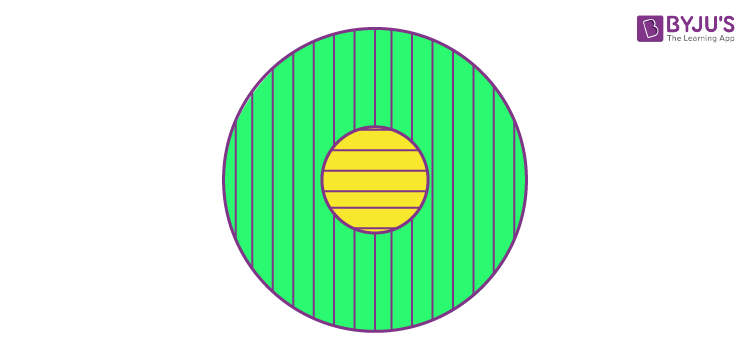Let I0 = intensity of the incident beam.

Then the intensity of the beam transmitted through the first Nicol prism is I1 = ½ I0

And through the 2nd prism is I2 = ½ I0 cos2φ

Through the Nth prism, it will be IN = IN-1 cos2φ

= ½ I0 cos2(N-1)φ

Hence fraction transmitted = IN/I0 = η = ½ cos(2N - 1)φ

= 0.12 for N = 6

And φ = 300

5. Natural light falls on a system of three identical in-line Polaroids, the principal direction of the middle Polaroid forming an angle φ = 600 with those of two other Polaroids. The maximum transmission coefficient of each Polaroid is equal to T = 0.81 when plane-polarized light falls on them. How many times will the intensity of the light decrease after its passing through the system?

Solution:

1. When natural light is incident on the first polaroid, the fraction transmitted will be ½ T (only the component polarized parallel to the principal direction of the polaroid will go).

The emergent light will be plane-polarized and on passing through the second Polaroid will be polarized in a different direction (corresponding to the principal direction of the 2nd polaroid) and the intensity will have decreased further by T cos2φ. In the third polaroid, the direction of polarization will again have to change by φ thus only a fraction T cos2φ will go through.

Finally

I = I0 × ½ T3 cos4φ

Thus the intensity will have decreased

I0/I = 2/T3cos4φ = 60.2 times

For T = 0.81, φ = 600.

6. The degree of polarization of partially polarized light is P = 0.25. Find the ratio of intensities of the polarized component of this light and the natural component.

Solution:

1. Suppose the partially polarized light consists of natural light of intensity l1 and plane-polarized light of intensity I2 with the direction of vibration parallel to, say, the x-axis. Then when a Polaroid is used to transmit it, the light transmitted will have a maximum intensity

½ I1 + I2

when the principal direction of the polaroid is parallel to x-axis, and will have a minimum intensity ½ I2 when the principal direction is perpendicular to the x-axis.

Thus P = (Imax-Imin)/(Imax+ Imin)

= I2/(I1 + I2)

I2/I1 = P/(1 - P)

= 0.25/0.75

= 1/3

7. A Nicol prism is placed in the way of partially polarized beam of light. When the prism is turned from the position of maximum transmission through an angle φ = 60°, the intensity of transmitted light decreased by a factor of η = 3.0. Find the degree of polarization of incident light.

Solution:

1. If, as above,

I1 = intensity of natural component

I2 = intensity of plane-polarized component

Then Imax = ½ I1 + I2

And I = Imax

= ½ I1 + I2 cos2φ

So I2 = Imax(1 - 1/η)cosec2φ

I1 = 2Imax (1 - (1 - 1/η)cosec2φ)

= (2Imax/sin2φ)(1/η - cos2φ)

Then P = I2/(I1 + I2)

= (1 - 1/η)/2(1/η - cos2φ) + 1 - 1/η

= (η - 1)/(1 - η cos 2φ)

On putting η = 3.0, φ = 600

We get P = 2/(1 + 3 ×1/2)

= ⅘

= 0.8

8. Natural light falls at the Brewster angle on the surface of glass. Using the Fresnel equations, find

(a) the reflection coefficient;

(b) the degree of polarization of refracted light.

Solution:

I’ = I sin21 - θ2)/sin21 + θ2)

And I’|| = I’||tan21 - θ2)/tan21 + θ2)

At the boundary between vacuum and a dielectric θ1 = θ2 since by Snell’s law

sin θ1 ≠ n sin θ2

Thus I’ /I cannot be zero. However if θ1 + θ2 = 900, I’|| = 0 and the reflected light is polarized in this case. The condition for this is

sin θ1 = n sin θ2, = n sin(90 - θ1)

tan θ1 = n θ1 is called Brewster’s angle.

The angle between reflected light and refracted light is 900 in this case.

9. A plane beam of natural light with intensity l0 falls on the surface of water at the Brewster angle. A fraction ρ = 0.039 of luminous flux is reflected. Find the intensity of the refracted beam.

Solution:

1.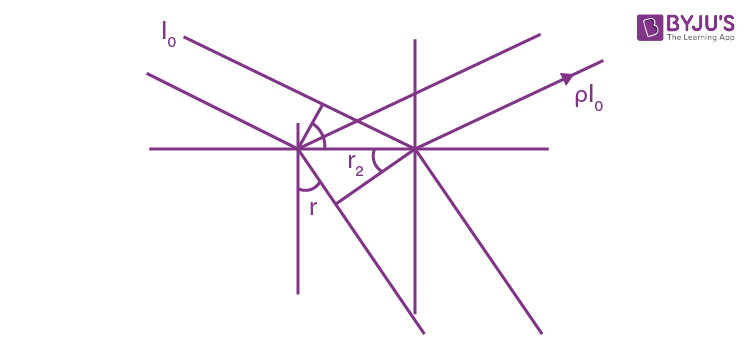The energy transmitted is, by conservation of energy, the difference between incident energy and the reflected energy. However, the intensity is affected by the change of the cross-section of the beam by refraction. Let Ai, Ar, At be the cross-sections of the incident, reflected and transmitted beams. Then

Then Ai = Ar

At = Ai cos r/cos i

But at Brewster’s angle r = 90 - i

So At = Ai tan i = nAi

Thus It = (1 - ρ)Io/n

10. A beam of plane-polarized light falls on the surface of water at the Brewster angle. The polarization plane of the electric vector of the electromagnetic wave makes an angle φ = 450 with the incidence plane. Find the reflection coefficient.

Solution:

1. The amplitude of the incident component whose oscillation vector is perpendicular to the plane of incidence is A = A0 sin φ

And similarly A|| = A0 cos φ

Then I’ = I0 [sin21 - θ2)/sin21 + θ2)] sin2φ

= I0 [(sinθ1 cos θ2 - cosθ1 sinθ2)/(sinθ1 cos θ2 + cosθ1 sinθ2)]2 sin2φ

= I0[(n2 - 1)/(n2 + 1)]2sin2φ

Hence ρ = I’/I0

= [(n2 - 1)/(n2 + 1)]2sin2φ

Putting n = 1.33 for water we get ρ = 0.0386

11. A narrow beam of natural light falls on the surface of a thick transparent plane-parallel plate at the Brewster angle. As a result, a fraction ρ = 0.080 of luminous flux is reflected from its top surface. Find the degree of polarization of beams 1-4 (Fig. 5.31)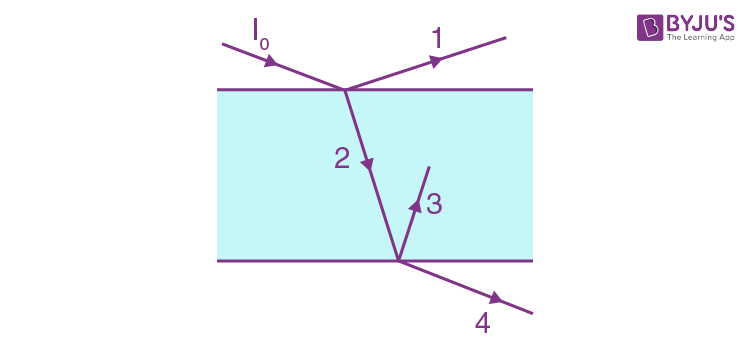Solution:

1. Since natural light is incident at Brewster’s angle, the reflected light 1 is completely polarized and P1 = 1. Similarly, the ray 2 is incident on glass air surface at Brewster’s angle (tan-11/n) so 3 is also completely polarized. Thus P3 = 1

Now P2 = ρ/(1 - ρ)

= 0.087 if ρ = 0.080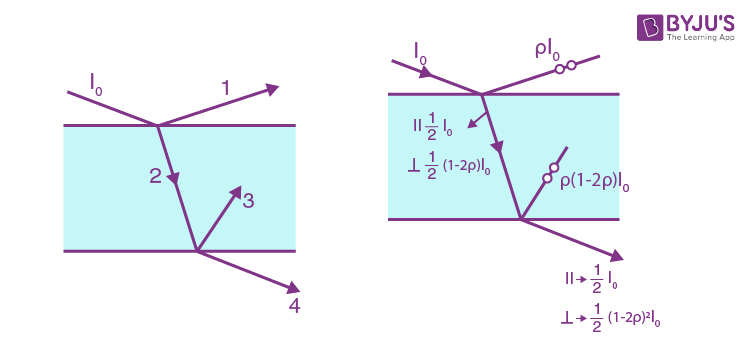Finally as shown in the figure

P4 = (½ - ½ (1 - 2ρ)2)/(½ + ½ (1 - 2ρ)2)

= 2ρ(1 - ρ)/(1 - 2ρ(1 - ρ)

= 0.173

12. A narrow beam of light of intensity l0 falls on a plane parallel glass plate (Fig. 5.31) at the Brewster angle. Using the Fresnel equations, find:

(a) the intensity of the transmitted beam I4 if the oscillation plane of the incident plane-polarized light is perpendicular to the incidence plane;

(b) the degree of polarization of the transmitted light if the light falling on the plate is natural.

Solution:

1. (a) In this case from Fresnel equations

I’ = I sin21 - θ2)/sin21 + θ2)

We get I1 = I0(n2 - 1)2/(n2 + 1)

= ρI0 (say)

Then I2 = (1 - ρ)I0

I3 = ρ(1 - ρ)I0

(ρ is invariant under the substitution n →1/n)

Finally I4 = (1 - ρ)2I0

= 16I0n4/(n2 + 1)4

= 0.726 I0

(b) Suppose ρ’ = coefficient of reflection for the component of light whose electric vector oscillates at right angles to the incidence plane.

From Fresnel's equations ρ’ = [(n2 - 1)/(n2+ 1)]2

Then in the transmitted beam we have a partially polarized beam which is a superposition of two (parallel and perpendicular) components with intensities

½ I0 and ½ I0(1 - ρ’)2

Thus P = (1 - (1 - ρ’)2)/(1 + (1 - ρ’)2)

= [(n2 + 1)4 - 16n4]/[(n2 + 1)4 + 16n4]

= (1 - 0.726)/(1 + 0.726)

≈ 0.158

13. A narrow beam of natural light falls on a set of N thick plane parallel glass plates at the Brewster angle. Find:

(a) the degree P of polarization of the transmitted beam;

(b) what P is equal to when N = 1, 2, 5, and 10.

Solution:

1. When natural light is incident on a glass plate at Brewster’s angle, the transmitted light has

I||’’ = I0/2

And I’’= [16n4/(n2 + 1)4]I0/2

= α4I0/2 where I0 is the incident intensity.

After passing through the 2nd plate, we find

I||’’’’ = ½ I0 and I|’’’’ = (α4)21/2 I0

Thus after N plates I||trans = ½ I0

Itrans = α4N ½ I0

Hence P = (1 - α4N)/(1 + α4N)

Where α = 2n/(1 + n2)

(b) α4 = 0.726 for n = 3/2

Thus P(N = 1) = 0.158, P(N = 2) = 0.310

P(N = 5) = 0.663, P(N = 10) = 0.922

14. Using Huygens's principle, construct the wave fronts and the propagation directions of the ordinary and extraordinary rays in a positive uniaxial crystal whose optical axis (a) is perpendicular to the incidence plane and parallel to the crystal;

(b) lies in the incidence plane and is parallel to the surface of the crystal;

(c) lies in the incidence plane at an angle of 450 to the surface of the crystal, and light falls at right angles to the optical axis.

Solution:

1.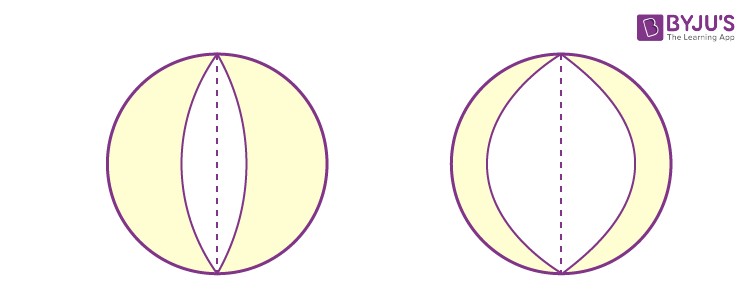The wave surface of a uniaxial crystal consists of two sheets of which one is a sphere while the other is an ellipsoid of revolution. The optic axis is the line joining the points of contact. To make the appropriate Huygens’s construction we must draw the relevant section of the wave surface inside the crystal and determine the directions of the ordinary and extraordinary rays. The result is as shown in Fig. 42 (a, b & c) of the answers.

15. A narrow beam of natural light with wavelength λ = 589 nm falls normally on the surface of a Wollaston polarizing prism made of Iceland spar as shown in Fig. 5.32. The optical axes of the two parts of the prism are mutually perpendicular. Find the angle δ between the directions of the beams behind the prism if the angle θ is equal to 300.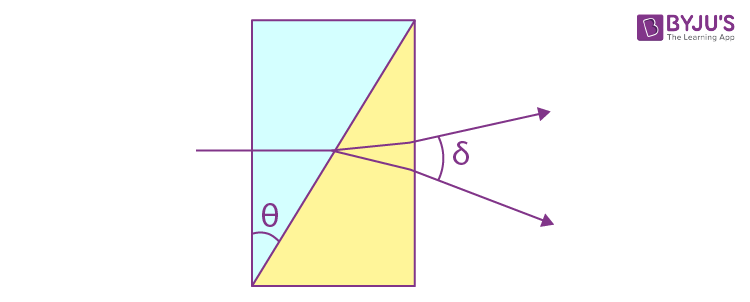Solution:

1. In a uniaxial crystal, an unpolarized beam of light (or even a polarized one) splits up into O (for ordinary) and E (for extraordinary) light waves. The direction of vibration in the O and E waves are most easily specified in terms of the O and E principal planes. The principal plane of the ordinary wave is defined as the plane containing the O ray and the optic axis.

Similarly the principal plane of the E wave is the plane containing the E ray and the optic axis. In terms of these planes the following is true : The O vibrations are perpendicular to the principal plane of the O ray while the E vibrations are in the principal plane of the E ray.

When we apply this definition to the Wollaston prism we find the following :

When unpolarized light enters from the left the O and E waves travel in the same direction but with different speeds. The O ray on the left has its vibrations normal to the plane of the paper and it becomes E ray on crossing the diagonal boundary of the two prisms similarly the E ray on the left becomes O ray on the right. In this case Snell’s law is applicable only approximately. The two rays are incident on the boundary at an angle θ and in the right prism the ray which we have called O ray on the right emerges at

sin-1(ne/n0) sin θ = sin-1(1.658/1.486)×1/2 = 33.910

Where we have used

ne = 1.1658, n0 = 1.486 and θ = 300

Similarly the E ray on the right emerges within the prism at

sin-1(n0/ne) sin θ = 26.620

This means that the O ray is incident at the boundary between the prism and air at

33.91 - 300 = 3.910

And will emerge into air with a deviation of sin-1(n0 sin 3.910) = sin-1(1.658 sin 3.910)

= 6.490

The E ray will emerge with an opposite deviation of sin-1(ne sin (300 - 26.620))

= sin-1(1.486 sin 3.380) = 5.030

Hence δ ≈ 6.490+5.030

= 11.520

This result is accurate to first order in (ne - n0) because Snell’s law holds when ne = n0.

16. A quartz plate cut parallel to the optical axis is placed between two crossed Nicol prisms. The angle between the principal directions of the Nicol prisms and the plate is equal to 450. The thickness of the plate is d = 0.50 mm. At what wavelengths in the interval from 0.50 to 0.60 μm is the intensity of light which passed through that system independent of rotation of the rear prism? The difference of refractive indices for ordinary and extraordinary rays in that wavelength interval is assumed to be ∆n = 0.0090.

Solution:

1. The quartz plate introduces a phase difference δ between the O and E components. When δ = π/2 (modulo π) the resultant wave is circularly polarized.

In this case intensity is independent of the rotation of the rear prism. Now

δ = (2π/λ)(ne - n0)d

= (2π/λ)(0.009 ×0.5×10-3 m

= 9π/λ, λ in μm.

For λ = 0.50 μm, δ = 18π. The relevant values of δ have to be chosen in the form

(k + ½) π. For k = 17, 16, 15 we get

λ = 0.5143 μm, 0.5435 μm and 0.5806 μm

These are the values of λ which lie between 0.50 μm and 0.60 μm.

17. White natural light falls on a system of two crossed Nicol prisms having between them a quartz plate 1.50 mm thick, cut parallel to the optical axis. The axis of the plate forms an angle of 450 with the principal directions of the Nicol prisms. The light transmitted through that system was split into the spectrum. How many dark fringes will be observed in the wavelength interval from 0.55 to 0.66 μm? The difference of refractive indices for ordinary and extraordinary rays in that wavelength interval is assumed to be equal to 0.0090.

Solution:

1. As in the previous two problems the quartz plate will introduce a phase difference δ. The light on passing through the plate will remain plane polarized only for δ = 2kπ or (2k + 1)π. In the latter case the plane of polarization of the light incident on the plate will be rotated by 90° by it so light passing through the analyzer (which was originally crossed) will be a maximum. Thus dark bands will be observed only for those λ for which

δ = 2kπ

Now δ = (2π/λ)(ne - n0)d

= (2π/λ) ×0.009 × 1.5×10-3 m

= (27π/λ) (λ in μm)

For λ = 0.55 we get δ = 49.09 π

Choosing δ = 48 π, 46 π, 44π, 42 π we get λ = 0.5625 μm, λ = 0.5870 μm, λ = 0.6136 μm, and λ = 0.6429 μm. These are the only values between 0.55 μm and 0.66 μm. Thus there are four bands.

18. A crystalline plate cut parallel to its optical axis is 0.25 mm thick and serves as a quarter-wave plate for a wavelength λ = 530 nm. At what other wavelengths of visible spectrum will it also serve as a quarter-wave plate? The difference of refractive indices for extraordinary and ordinary rays is assumed to be constant and equal to ne - no = 0 .0090 at all wavelengths of the visible spectrum.

Solution:

1. Here δ = (2π/λ) × 0.009×0.25 m

= 4.5π/λ, λ in μm.

We check for that λ = 428.6 nm, δ = 10.5 π

λ = 529.4 nm, δ = 8.5 π

λ = 692.3 nm, δ = 6.5 π

These are the only values of λ, for which the plate acts as a quarter wave plate.

19. Light passes through a system of two crossed Nicol prisms between which a quartz plate cut at right angles to its optical axis is placed. Determine the minimum thickness of the plate which allows light of wavelength 436 nm to be completely cut off by the system and transmits half the light of wavelength 497 nm. The specific rotation constant of quartz for these wavelengths is equal to 41.5 and 31.1 angular degrees per mm respectively.

Solution:

1. For light of wavelength 436 nm

41.50×d = k×1800

= 2k×900

(Light will be completely cut off when the quartz plate rotates the plane of polarization by a multiple of 1800.) Here d = thickness of quartz plate in mm. For natural incident light, half the light will be transmitted when the quartz rotates light by an odd multiple of 900. Thus

31.10×d = (2k’ + 1)×900

Now 41.5/31.1 = 1.3344 ≈ 4/3

Thus k = 2 and k’ = 1 and

d = 4×90/41.5

= 8.67 mm

20. Plane-polarized light of wavelength 589 nm propagates along the axis of a cylindrical glass vessel filled with slightly turbid sugar solution of concentration 500 g/l. Viewing from the side, one can see a system of helical fringes, with 50 cm between neighbouring dark fringes along the axis. Explain the emergence of the fringes and determine the specific rotation constant of the solution.

Solution:

1.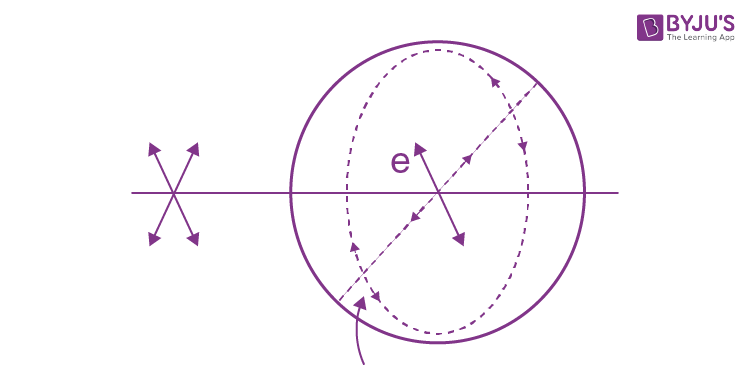Two effects are involved here : rotation of plane of and the polarization by sugar solution and the effect of that rotation on the scattering of light in the transverse direction. The latter is shown in the figure given below. It is easy to see trom the figure that there will be no scattering of light in this transverse direction if the incident light has its electric vector parallel to the line of sight. In such a situation, we expect fringes to occur in the given experiment. From the given data we see that in a distance of 50 cm, the rotation of plane of polarization must be 1800. Thus the specific rotation constant of sugar

= rotation constant/concentration

= [(180/50)/(500 g/l)]ang/deg/cm

= 180/(5.0 dm × 0.500 gm/cc)

= 720 ang deg/(dm. gm/cc) (1 dm = 10 cm).